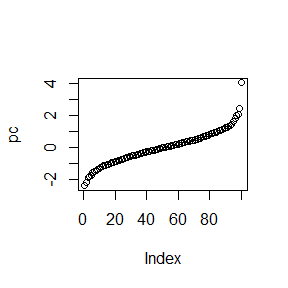# vtable Bonus Functions

#### 2022-07-14

The vtable package serves the purpose of outputting automatic variable documentation that can be easily viewed while continuing to work with data.

vtable contains four main functions: vtable() (or vt()), sumtable() (or st()), labeltable(), and dftoHTML()/dftoLaTeX().

This vignette focuses on some bonus helper functions that come with vtable that have been exported because they may be handy to you. This can come in handy for saving a little time, and can help you avoid having to create an unnamed function when you need to call a function.

# Shortcut Helper Functions

vtable includes four shortcut functions. These are generally intended for use with the summ option in vtable and sumtable because nested functions don’t look very nice in a vtable, or in a sumtable unless you explicitly set the summ.names.

## nuniq

nuniq(x) returns length(unique(x)), the number of unique values in the vector.

## countNA, propNA, and notNA

These three functions are shortcuts for dealing with missing data. You have probably written out the nested versions of these many times!

Function Short For
countNA() sum(is.na())
propNA() mean(is.na())
notNA() sum(!is.na())

## is.round

This function is a shortcut for !any(!(x == round(x,digits))).

It takes two arguments: a vector x and a number of digits (0 by default). It checks whether you can round to digits digits without losing any information.

# Other Helper Functions

## pctile

pctile(x) is short for quantile(x,1:100/100). So in one sense this is another shortcut function. But this inherently lets you interact with percentiles a bit differently.

While quantile() has you specify which percentile you want in the function call, pctile() returns an object with all integer percentiles, and you can pull out which ones you want afterwards. pctile(x) is the 50th percentile, etc.. This can be convenient in several applications, an obvious one being in sumtable.

library(vtable)
#Some random normal data, and its percentiles
d <- rnorm(1000)
pc <- pctile(d)

#25th, 50th, 75th percentile
pc[c(25,50,75)]
##          25%          50%          75%
## -0.698256183  0.005340094  0.661151636
#Inverse normal CDF with 100 points of articulation
plot(pc)## weighted.sd

weighted.sd(x, w) is a function to calculate a weighted standard deviation of x using w as weights, much like the base weighted.mean() does for means. It is mostly used as a helper function for sumtable() when group.weights is specified. However, you can use it on its own if you like. Unlike weighted.mean(), setting na.rm = TRUE will account for missings both in x and w.

The weighted standard deviation is calculated as

$\frac{\sum_i(w_i*(x_i-\bar{x}_w)^2)}{\frac{N_w-1}{N_w}\sum_iw_i}$

Where $$\bar{x}_w$$ is the weighted mean of $$x$$, and $$N_w$$ is the number of observations with a nonzero weight.

x <- 1:100
w <- 1:100
weighted.mean(x, w)
##  67
sd(x)
##  29.01149
weighted.sd(x, w)
##  23.80476

# independence.test

independence.test is a helper function for sumtable(group.test=TRUE) that tests for independence between a categorical variable x and another variable y that may be categorical or numerical.

Then, it outputs a formatted string as its output, with significance stars, for printing.

The function takes the format

independence.test(x,y,w=NA,
factor.test = NA,
numeric.test = NA,
star.cutoffs = c(.01,.05,.1),
star.markers = c('***','**','*'),
digits = 3,
fixed.digits = FALSE,
format = '{name}={stat}{stars}',
opts = list())

## factor.test and numeric.test

These are functions that actually perform the independence test. numeric.test is used when y is numeric, and factor.test is used in all other instances.

Specifically, these functions should take only x, y, and w=NULL as arguments, and should return a list with three elements: the name of the test statistic, the test statistic itself, and the p-value of the test.

By default, these are the internal functions vtable:::chisq.it for factor.test and vtable:::groupf.it for numeric.test, so you can take a look at those (just put vtable:::chisq.it in the terminal and it will show you the function’s code) if you’d like to make your own test functions.

## star.cutoffs and star.markers

These are numeric and character vectors, respectively, used for p-value cutoffs and to create significance markers.

star.cutoffs indicates the cutoffs, and star.markers indicates the markers to be used with each cutoff, in the same order. So with star.cutoffs = c(.01,.05,.1) and star.markers = c('***','**','*'), each p-value below .01 will get marked with '***', each from .01 to .05 will get '**', and each from .05 to .1 will get *.

Defaults are set to “economics defaults” (.1, .05, .01). But these are of course easy to change.

data(iris)
independence.test(iris$Species, iris$Sepal.Length,
star.cutoffs = c(.05,.01,.001))
##  "F=119.265*"

## digits and fixed.digits

digits indicates how many digits after the decimal place from the test statistics and p-values should be displayed. fixed.digits determines whether trailing zeros are maintained.

independence.test(iris$Species, iris$Sepal.Width,
digits=1)
##  "F=49.2***"
independence.test(iris$Species, iris$Sepal.Width,
digits=4,
fixed.digits = TRUE)
##  "F=49.1600***"

## format

This is the printing format that the output will produce, incorporating the name of the test statistic {name}, the test statistic {stat}, the significance markers {stars}, and the p-value {pval}.

If your independence.test is heading out to another format besides being printed in the R console, you may want to add additional markup like '{name}$={stat}^{stars}$'} in LaTeX or '{name}={stat}<sup>{stars}</sup>' in HTML. If you do this, be sure to think carefully about escaping or not escaping characters as appropriate when you print!

independence.test(iris$Species, iris$Sepal.Width,
format = 'Pr(>{name}): {pval}{stars}')
##  "Pr(>F): <0.001***"

## opts

You can create a named list where the names are the above options and the values are the settings for those options, and input it into independence.test using opts=. This is an easy way to set the same options for many independence.tests.